# (6)(20 points) (a) Let G be a cyclic group of order 72. Prove that for every...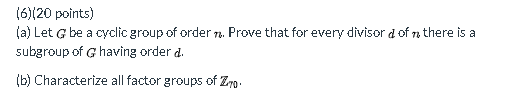(6)(20 points) (a) Let G be a cyclic group of order 72. Prove that for every divisor d of a there is a subgroup of G having order d. (b) Characterize all factor groups of Zyo.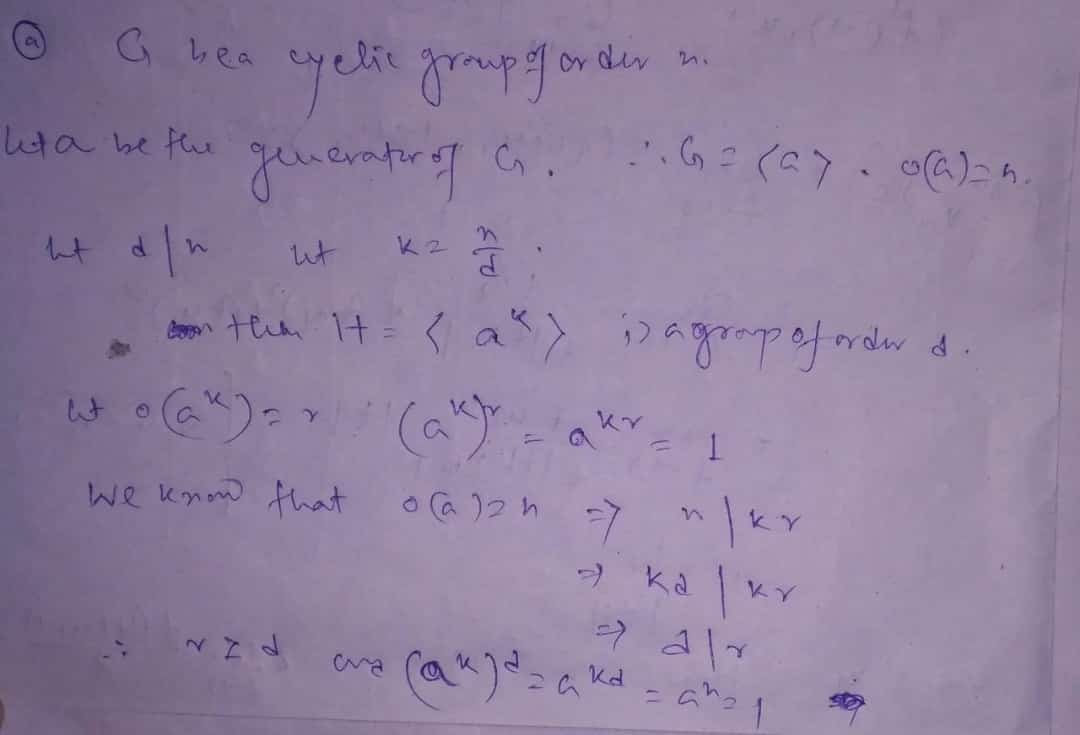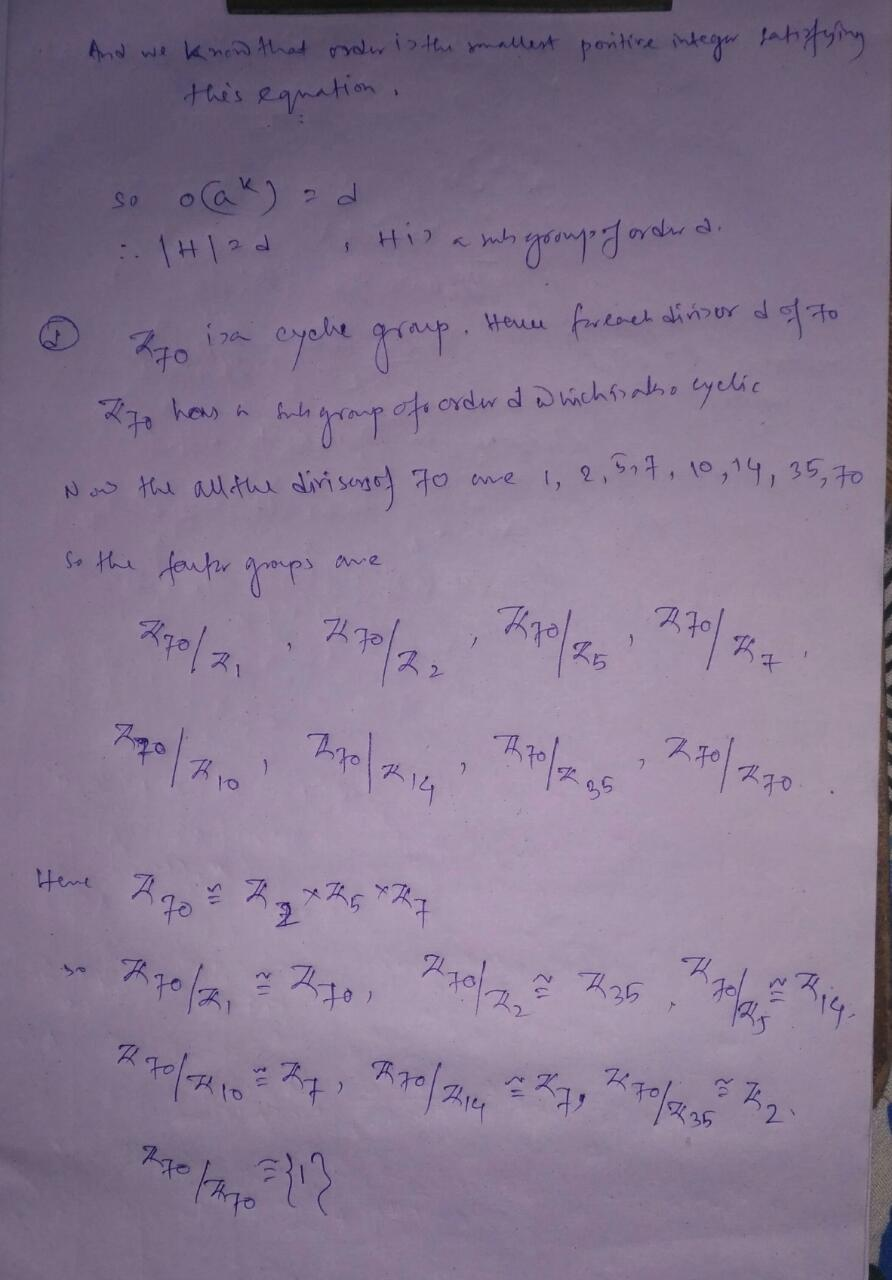##### Add Answer of: (6)(20 points) (a) Let G be a cyclic group of order 72. Prove that for every...
Similar Homework Help Questions
• ### (6)(20 points) (a) Let G be a cyclic group of order n. Prove that for every...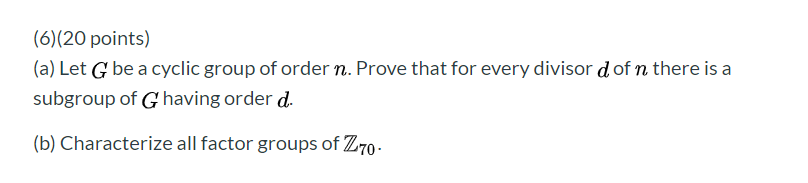(6)(20 points) (a) Let G be a cyclic group of order n. Prove that for every divisor d of n there is a subgroup of G having order d. (b) Characterize all factor groups of Z70 -

• ### (6)(20 points) (a) Let G be a cyclic group of order n. Prove that for every...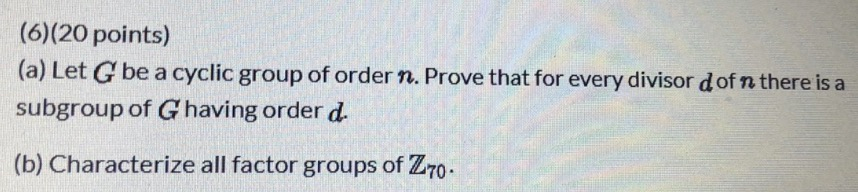(6)(20 points) (a) Let G be a cyclic group of order n. Prove that for every divisor dofn there is a subgroup of Ghaving order d. (b) Characterize all factor groups of Z70.

• ### ASAP PLEASE (6)(20 points) (a) Let G be a cyclic group of order n. Prove that...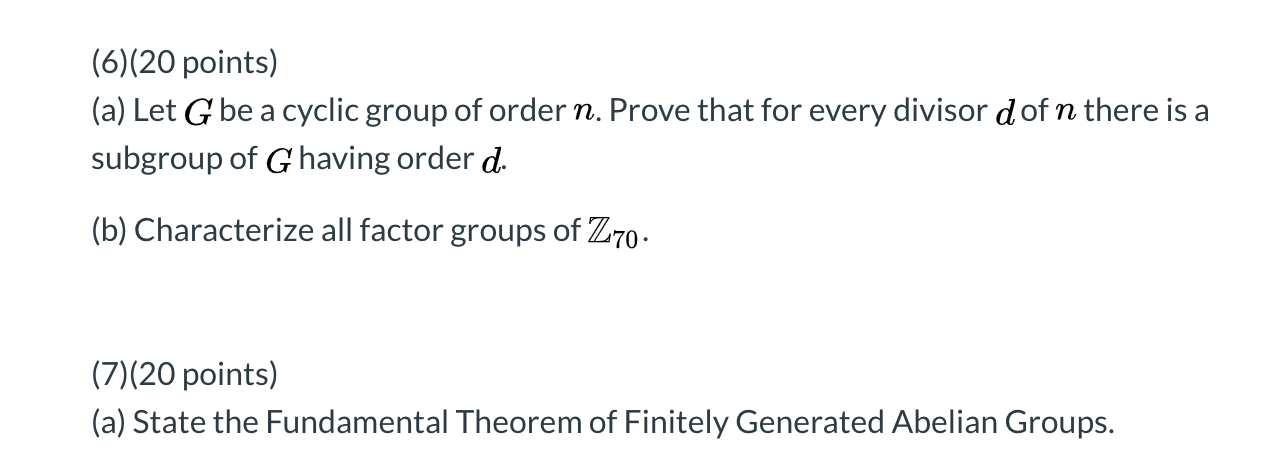ASAP PLEASE (6)(20 points) (a) Let G be a cyclic group of order n. Prove that for every divisor dof n there is a subgroup of G having order d. (b) Characterize all factor groups of Z70. (7)(20 points) (a) State the Fundamental Theorem of Finitely Generated Abelian Groups.

• ### (a) Let G be a cyclic group of order n. Prove that fo every divisor d...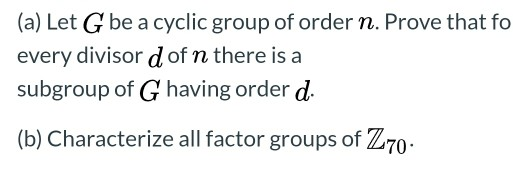(a) Let G be a cyclic group of order n. Prove that fo every divisor d of n there is a subgroup of G having order d. (b) Characterize all factor groups of Z70.

• ### (a) Let  be a cyclic group of order . Prove that for every divisor  of  there is a subgroup...

(a) Let  be a cyclic group of order . Prove that for every divisor  of  there is a subgroup of  having order . (b) Characterize all factor groups of

• ### need help ASAP with 6 and 7. Thank You! (6)(20 points) (a) Let G be a...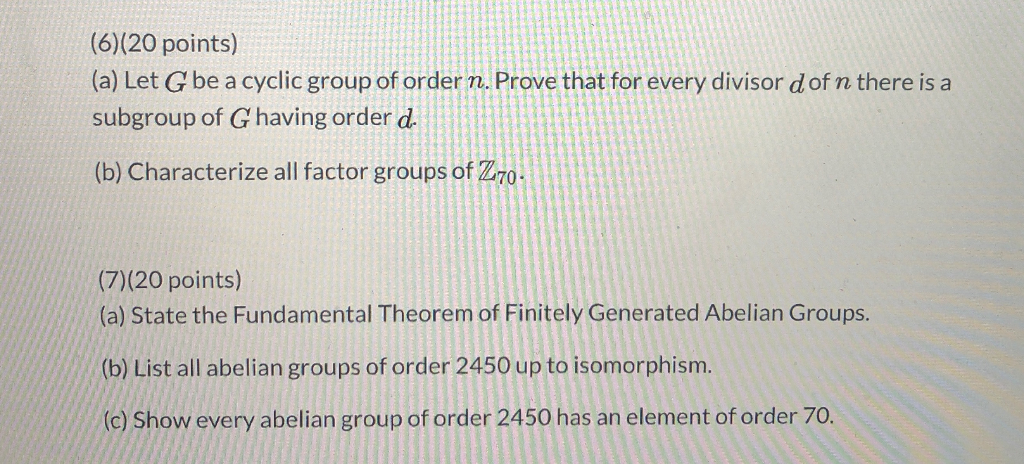need help ASAP with 6 and 7. Thank You! (6)(20 points) (a) Let G be a cyclic group of order n. Prove that for every divisor dof n there is a subgroup of Ghaving order d. (b) Characterize all factor groups of Z70. (7)(20 points) (a) State the Fundamental Theorem of Finitely Generated Abelian Groups. (b) List all abelian groups of order 2450 up to isomorphism. (c) Show every abelian group of order 2450 has an element of order 70.

• ### (5)(20 points) In the problems below, give the order of the element in the indicated factor...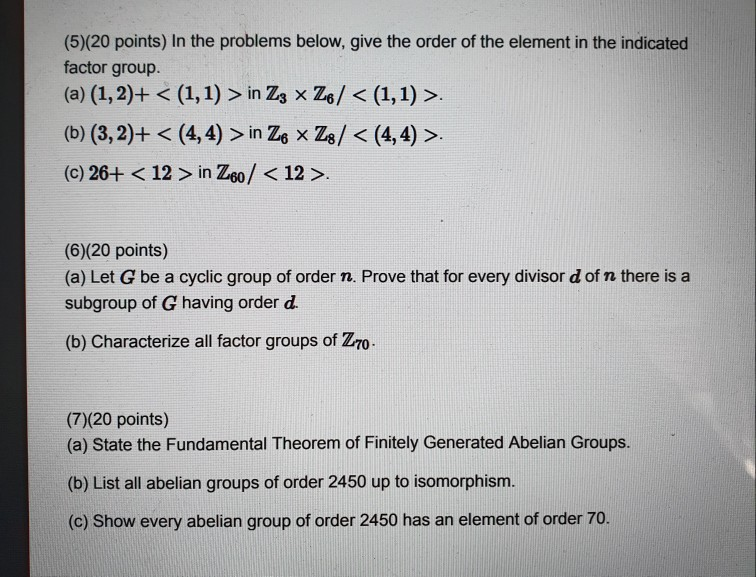(5)(20 points) In the problems below, give the order of the element in the indicated factor group. (a) (1, 2)+ < (1,1) > in Z3 x Z6/ < (1,1) >. (b) (3,2)+ < (4,4) > in Ze * Z8/ < (4,4) >. (c) 26+ < 12 > in Z60 / <12>. (6)(20 points) (a) Let G be a cyclic group of order n. Prove that for every divisor d of n there is a subgroup of G having order d....

• ### (6 points) Let G be a group of order 35. Show that every non-trivial subgroup of...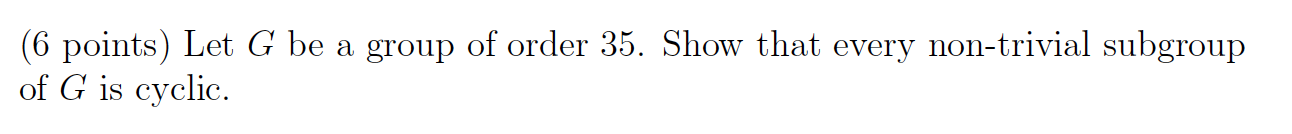(6 points) Let G be a group of order 35. Show that every non-trivial subgroup of G is cyclic.

• ### 8. (20 points) Let G Zs x Zg and let H be the cyclic subgroup generated by (3, 3). (a) Find the o...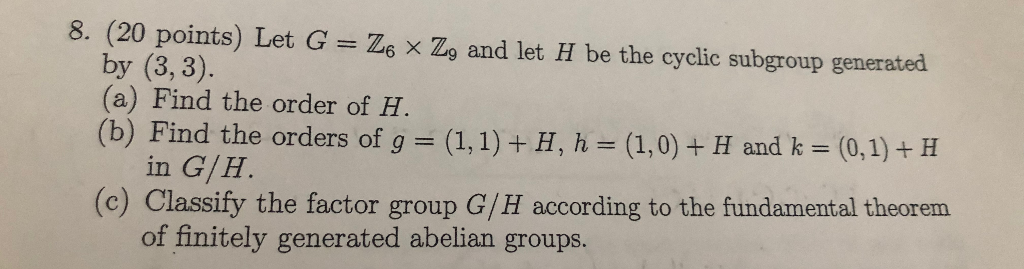8. (20 points) Let G Zs x Zg and let H be the cyclic subgroup generated by (3, 3). (a) Find the order of H (b) Find the orders of g = (1,1) + H, h = (1,0) + H and k = (0,1) + H in G/H (c) Classify the factor group G/H according to the fundamental theorem of finitely generated abelian groups. 8. (20 points) Let G Zs x Zg and let H be the cyclic subgroup generated...

• ### (a) Let G be a group of order 4 with identity e. Show that G is either cyclic or a2-e for all (b)...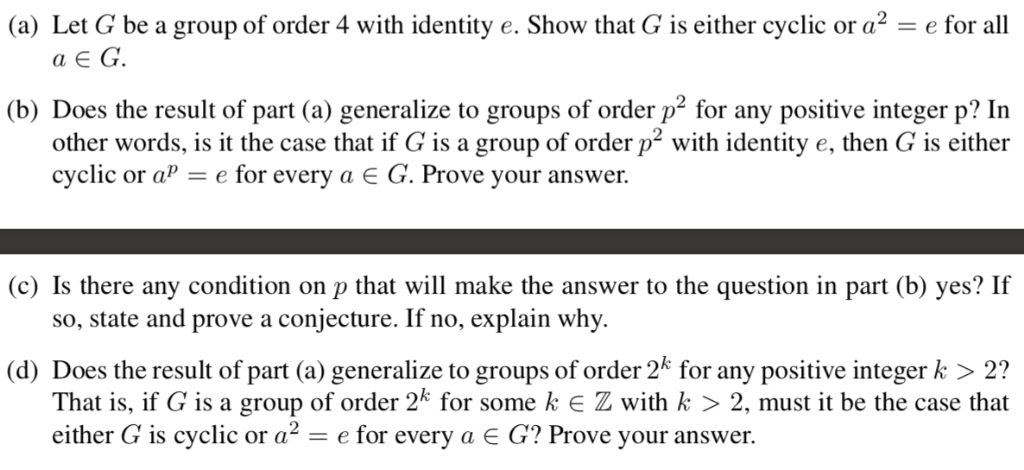(Abstract Algebra) Please answer a-d clearly. Show your work and explain your answer. (a) Let G be a group of order 4 with identity e. Show that G is either cyclic or a2-e for all (b) Does the result of part (a) generalize to groups of order p2 for any positive integer p? In other words, is it the case that if G is a group of order p2 with identity e, then is either cyclic or a- e for...

Free Homework App